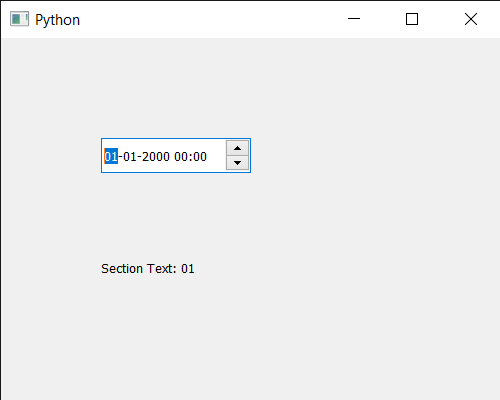Skip to content
Related Articles
PyQt5 QDateTimeEdit – Getting Section Text
• Last Updated : 14 Jul, 2020

In this article we will see how we can get the section text of the QDateTimeEdit widget. There are many sections in the QDateTimeedit widget like, year, month, hour, minute section. User can select any section with the help of mouse or keyboard and increment and decrement action get performed on the selected section. Section text is used to get the text of only the selected section.

In order to do this we will use `sectionText` method with the QDateTimeEdit object.

Syntax : datetimeedit.sectionText(section)

Argument : It takes section object as argument

Return : It returns string

Below is the implementation

 `# importing libraries``from` `PyQt5.QtWidgets ``import` `*` `from` `PyQt5 ``import` `QtCore, QtGui``from` `PyQt5.QtGui ``import` `*` `from` `PyQt5.QtCore ``import` `*` `import` `sys`` ` ` ` `class` `Window(QMainWindow):`` ` `    ``def` `__init__(``self``):``        ``super``().__init__()`` ` `        ``# setting title``        ``self``.setWindowTitle(``"Python "``)`` ` `        ``# setting geometry``        ``self``.setGeometry(``100``, ``100``, ``500``, ``400``)`` ` `        ``# calling method``        ``self``.UiComponents()`` ` `        ``# showing all the widgets``        ``self``.show()`` ` `    ``# method for components``    ``def` `UiComponents(``self``):`` ` `        ``# creating a QDateTimeEdit widget``        ``datetimeedit ``=` `QDateTimeEdit(``self``)`` ` `        ``# setting geometry``        ``datetimeedit.setGeometry(``100``, ``100``, ``150``, ``35``)`` ` ` ` `        ``# creating a label``        ``label ``=` `QLabel(``"GeeksforGeeks"``, ``self``)`` ` `        ``# setting geometry to the label``        ``label.setGeometry(``100``, ``200``, ``200``, ``60``)`` ` `        ``# making label multi line``        ``label.setWordWrap(``True``)`` ` `        ``# getting section at index 1``        ``section ``=` `datetimeedit.sectionAt(``1``)`` ` `        ``# getting section count``        ``value ``=` `datetimeedit.sectionText(section)`` ` `        ``# setting text to the label``        ``label.setText(``"Section Text: "` `+` `str``(value))`` ` `        ` ` ` `# create pyqt5 app``App ``=` `QApplication(sys.argv)`` ` `# create the instance of our Window``window ``=` `Window()`` ` `# start the app``sys.exit(App.``exec``())`

Output :Attention geek! Strengthen your foundations with the Python Programming Foundation Course and learn the basics.

To begin with, your interview preparations Enhance your Data Structures concepts with the Python DS Course. And to begin with your Machine Learning Journey, join the Machine Learning – Basic Level Course

My Personal Notes arrow_drop_up